# 使用编辑距离算法进行模糊匹配

``````import pandas as pd

excel = pd.ExcelFile("所有客户.xlsx")
data = excel.parse(0)
find = excel.parse(1)
print(data.shape)
print(find.shape)
``````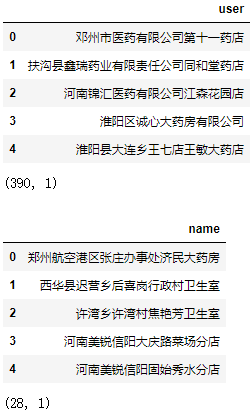``````def minDistance(word1: str, word2: str):
'编辑距离的计算函数'
n = len(word1)
m = len(word2)
# 有一个字符串为空串
if n * m == 0:
return n + m
# DP 数组
D = [ * (m + 1) for _ in range(n + 1)]
# 边界状态初始化
for i in range(n + 1):
D[i] = i
for j in range(m + 1):
D[j] = j
# 计算所有 DP 值
for i in range(1, n + 1):
for j in range(1, m + 1):
left = D[i - 1][j] + 1
down = D[i][j - 1] + 1
left_down = D[i - 1][j - 1]
if word1[i - 1] != word2[j - 1]:
left_down += 1
D[i][j] = min(left, down, left_down)
return D[n][m]
``````

``````result = []
for name in find.name.values:
a = data.user.apply(lambda user: minDistance(user, name))
user = data.user[a.argmin()]
result.append(user)
find["result"] = result
find
``````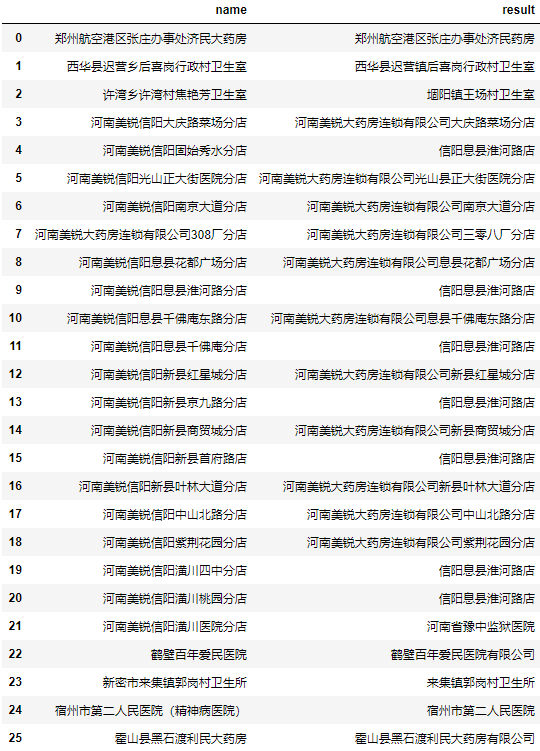``````a = data.user.apply(lambda user: minDistance(user, '河南美锐信阳息县淮河路分店'))
a = a.nsmallest(10).reset_index()
a.columns = ["名称", "编辑距离"]
a.名称 = data.user[a.名称].values
a
``````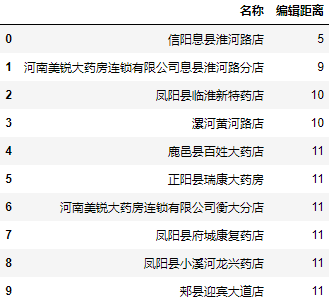``````a = data.user.apply(lambda user: minDistance(user, '河南美锐信阳潢川四中分店'))
a = a.nsmallest(10).reset_index()
a.columns = ["名称", "编辑距离"]
a.名称 = data.user[a.名称].values
a
``````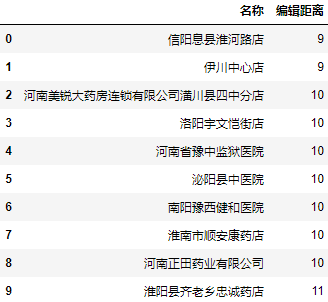# 使用fuzzywuzzy进行批量模糊匹配

## fuzz模块

``````from fuzzywuzzy import fuzz
``````

``````a = data.user.apply(lambda user: fuzz.ratio(user, '河南美锐信阳潢川四中分店'))
a = a.nlargest(10).reset_index()
a.columns = ["名称", "相似度"]
a.名称 = data.user[a.名称].values
a
``````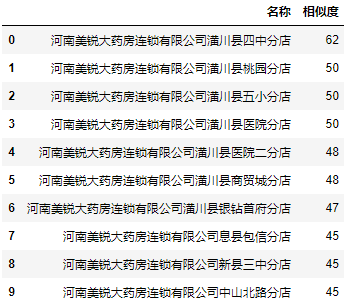``````a = data.user.apply(lambda user: fuzz.partial_ratio(user, '河南美锐信阳潢川四中分店'))
a = a.nlargest(10).reset_index()
a.columns = ["名称", "相似度"]
a.名称 = data.user[a.名称].values
a
``````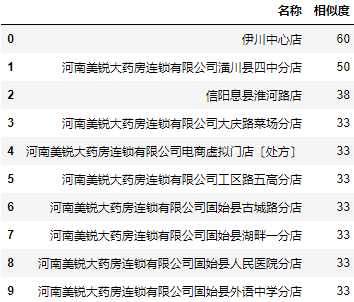## process模块

process模块则是进一步的封装，可以直接获取相似度最高的值和相似度：

``````from fuzzywuzzy import process
``````

extract提取多条数据：

``````users = data.user.to_list()
a = process.extract('河南美锐信阳潢川四中分店', users, limit=10)
a = pd.DataFrame(a, columns=["名称", "相似度"])
a
``````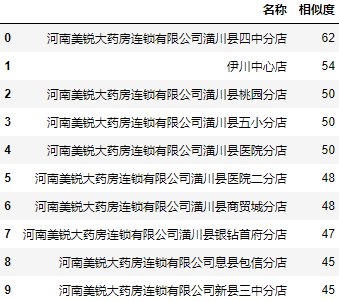``````users = data.user.to_list()
find["result"] = find.name.apply(lambda x: process.extractOne(x, users))
find
``````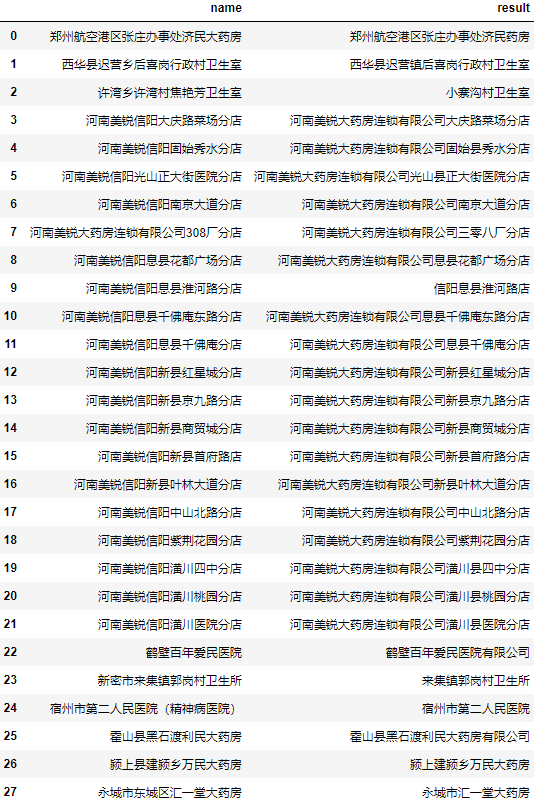``````process.extract('许湾乡许湾村焦艳芳卫生室', users)
``````
``````[('小寨沟村卫生室', 51),
('周口城乡一体化焦艳芳一体化卫生室', 50),
('西华县皮营乡楼陈村卫生室', 42),
('叶县邓李乡杜杨村第二卫生室', 40),
('汤阴县瓦岗乡龙虎村东卫生室', 40)]
``````
``````process.extract('河南美锐信阳息县淮河路分店', users)
``````
``````[('信阳息县淮河路店', 79),
('河南美锐大药房连锁有限公司息县淮河路分店', 67),
('河南美锐大药房连锁有限公司息县大河文锦分店', 53),
('河南美锐大药房连锁有限公司息县千佛庵东路分店', 51),
('河南美锐大药房连锁有限公司息县包信分店', 50)]
``````

``````result = find.name.apply(lambda x: next(zip(*process.extract(x, users, limit=3)))).apply(pd.Series)
result.rename(columns=lambda i: f"匹配{i+1}", inplace=True)
result = pd.concat([find.drop(columns="result"), result], axis=1)
result
``````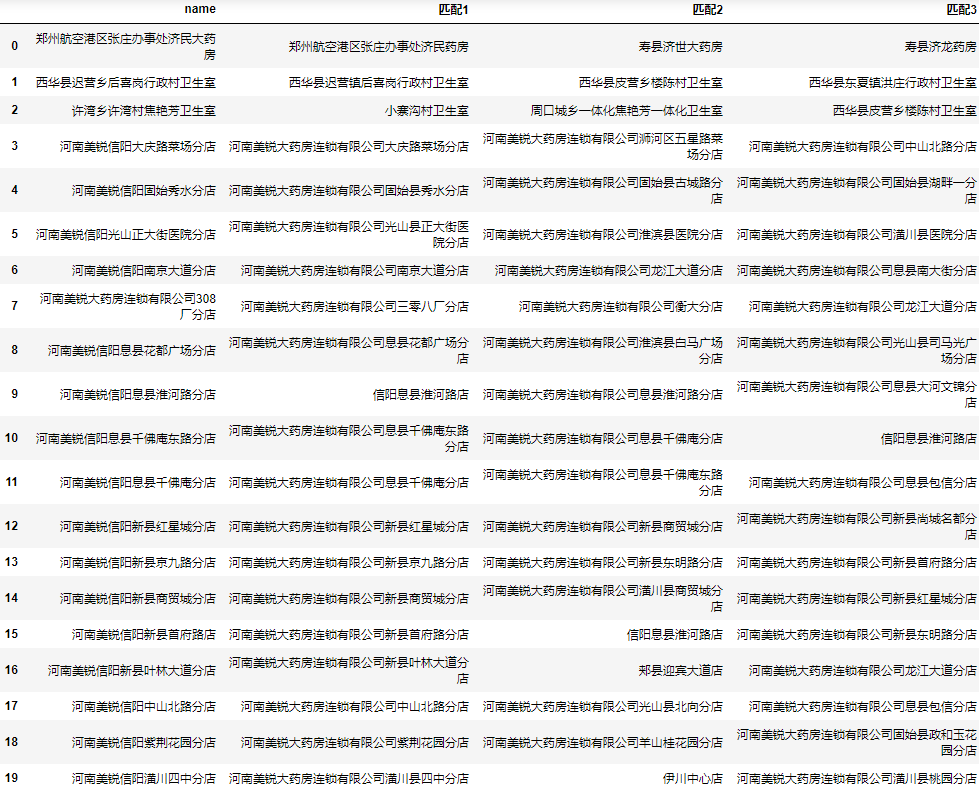## 整体代码

``````from fuzzywuzzy import process
import pandas as pd

excel = pd.ExcelFile("所有客户.xlsx")
data = excel.parse(0)
find = excel.parse(1)
users = data.user.to_list()
result = find.name.apply(lambda x: next(
zip(*process.extract(x, users, limit=3)))).apply(pd.Series)
result.rename(columns=lambda i: f"匹配{i+1}", inplace=True)
result = pd.concat([find, result], axis=1)
result
``````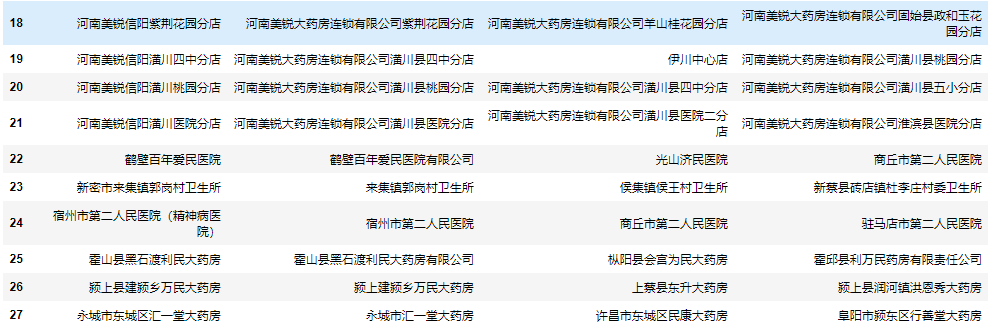# 使用Gensim进行批量模糊匹配

## Gensim简介

Gensim支持包括TF-IDF，LSA，LDA，和word2vec在内的多种主题模型算法，支持流式训练，并提供了诸如相似度计算，信息检索等一些常用任务的API接口。

• 语料（Corpus）：一组原始文本的集合，用于无监督地训练文本主题的隐层结构。语料中不需要人工标注的附加信息。在Gensim中，Corpus通常是一个可迭代的对象（比如列表）。每一次迭代返回一个可用于表达文本对象的稀疏向量。
• 向量（Vector）：由一组文本特征构成的列表。是一段文本在Gensim中的内部表达。
• 稀疏向量（SparseVector）：可以略去向量中多余的0元素。此时，向量中的每一个元素是一个(key, value)的元组
• 模型（Model）：是一个抽象的术语。定义了两个向量空间的变换（即从文本的一种向量表达变换为另一种向量表达）。

``````import pandas as pd

print(data.shape)
print(find.shape)
``````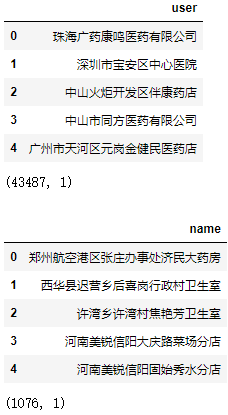## 使用词袋模型直接进行批量相似度匹配

``````import jieba

data_split_word = data.user.apply(jieba.lcut)
``````
``````0        [珠海, 广药, 康鸣, 医药, 有限公司]
1              [深圳市, 宝安区, 中心医院]
2         [中山, 火炬, 开发区, 伴康, 药店]
3           [中山市, 同方, 医药, 有限公司]
4    [广州市, 天河区, 元岗金, 健民, 医药, 店]
5       [广州市, 天河区, 元岗居, 健堂, 药房]
6          [广州市, 天河区, 元岗润佰, 药店]
7        [广州市, 天河区, 元岗, 协心, 药房]
8        [广州市, 天河区, 元岗, 心怡, 药店]
9         [广州市, 天河区, 元岗永亨堂, 药店]
Name: user, dtype: object
``````

``````from gensim import corpora

dictionary = corpora.Dictionary(data_split_word.values)
data_corpus = data_split_word.apply(dictionary.doc2bow)
``````
``````0             [(0, 1), (1, 1), (2, 1), (3, 1), (4, 1)]
1                             [(5, 1), (6, 1), (7, 1)]
2          [(8, 1), (9, 1), (10, 1), (11, 1), (12, 1)]
3                   [(0, 1), (3, 1), (13, 1), (14, 1)]
4    [(0, 1), (15, 1), (16, 1), (17, 1), (18, 1), (...
Name: user, dtype: object
``````

``````from gensim import similarities

index = similarities.SparseMatrixSimilarity(data_corpus.values, num_features=len(dictionary))
``````

``````find_corpus = find.name.apply(jieba.lcut).apply(dictionary.doc2bow)
sim = index[find_corpus]
find["result"] = data.user[sim.argmax(axis=1)].values
``````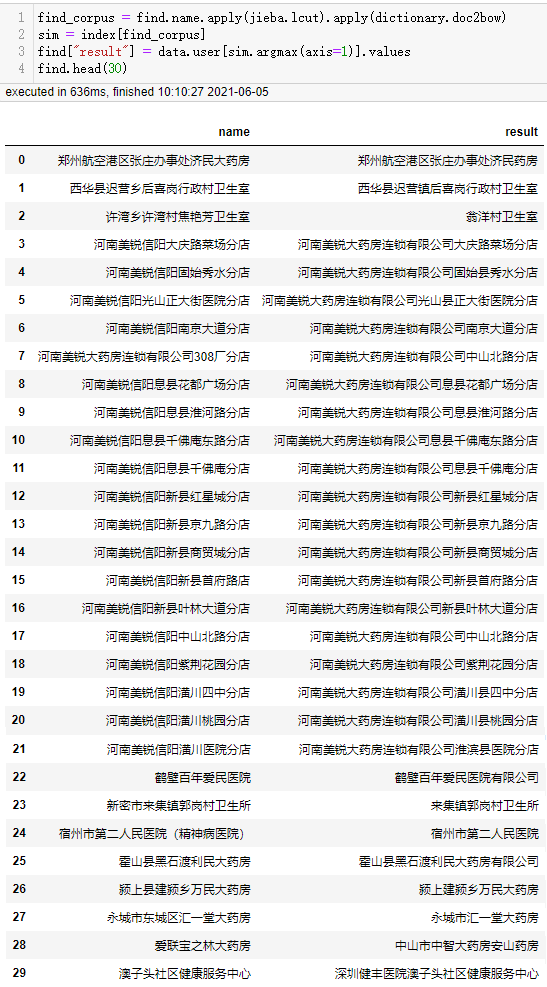## 使用TF-IDF主题向量变换后进行批量相似度匹配

``````from gensim import models

tfidf = models.TfidfModel(data_corpus.to_list())
index = similarities.SparseMatrixSimilarity(
tfidf[data_corpus], num_features=len(dictionary))
``````

``````sim = index[tfidf[find_corpus]]
find["result"] = data.user[sim.argmax(axis=1)].values
``````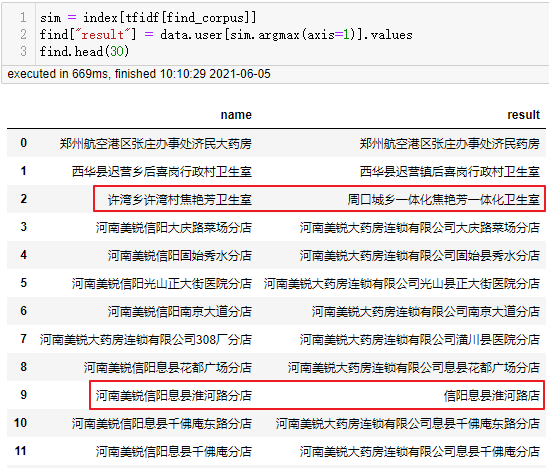``````from gensim import models

tfidf = models.TfidfModel(data_corpus.to_list())
index = similarities.SparseMatrixSimilarity(
tfidf[data_corpus], num_features=len(dictionary))
sim = index[find_corpus]
find["result"] = data.user[sim.argmax(axis=1)].values
``````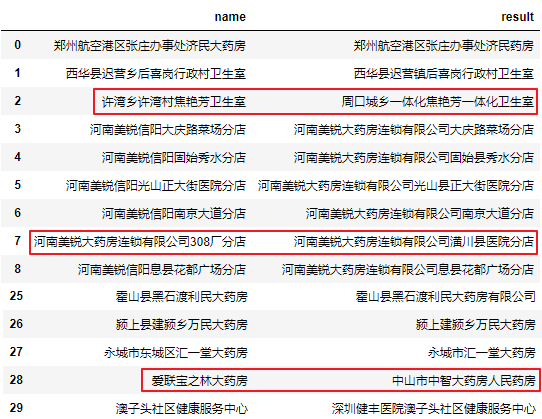``````trantab = str.maketrans("0123456789", "零一二三四五六七八九")
find_corpus = find.name.apply(lambda x: dictionary.doc2bow(jieba.lcut(x.translate(trantab))))

sim = index[find_corpus]
find["result"] = data.user[sim.argmax(axis=1)].values
``````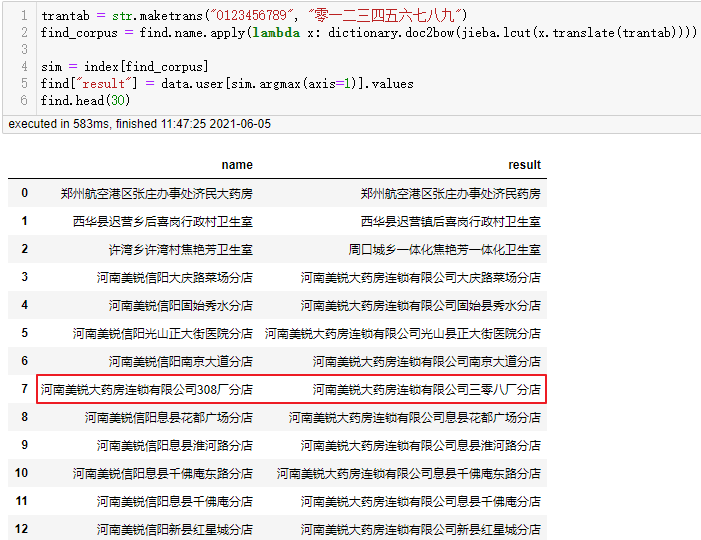## 同时获取最大的3个结果

``````result = []
for corpus in find_corpus.values:
sim = pd.Series(index[corpus])
result.append(data.user[sim.nlargest(3).index].values)
result = pd.DataFrame(result)
result.rename(columns=lambda i: f"匹配{i+1}", inplace=True)
result = pd.concat([find.drop(columns="result"), result], axis=1)
``````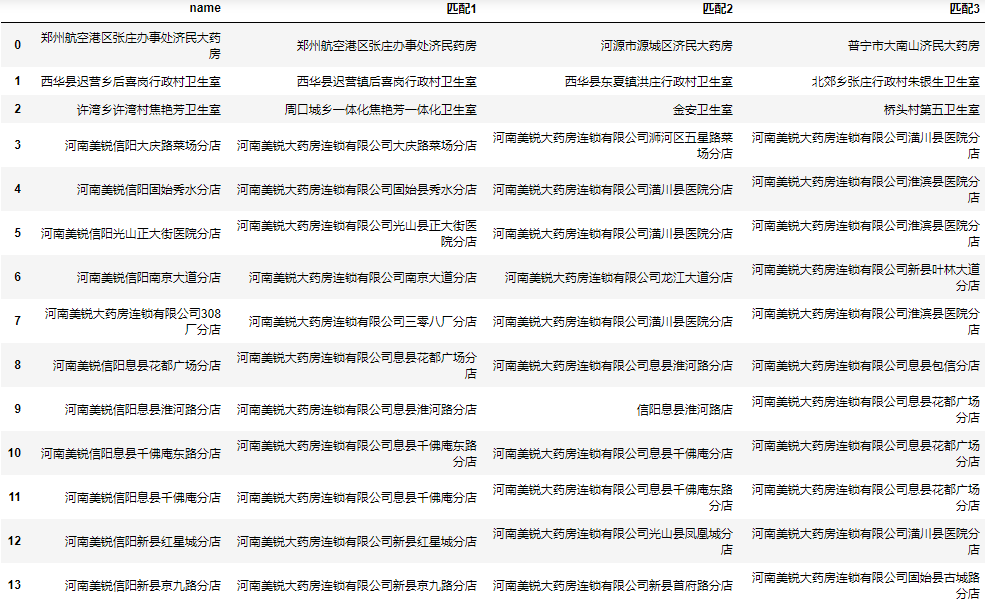## 完整代码

``````from gensim import corpora, similarities, models
import jieba
import pandas as pd

data_split_word = data.user.apply(jieba.lcut)
dictionary = corpora.Dictionary(data_split_word.values)
data_corpus = data_split_word.apply(dictionary.doc2bow)
trantab = str.maketrans("0123456789", "零一二三四五六七八九")
find_corpus = find.name.apply(
lambda x: dictionary.doc2bow(jieba.lcut(x.translate(trantab))))

tfidf = models.TfidfModel(data_corpus.to_list())
index = similarities.SparseMatrixSimilarity(
tfidf[data_corpus], num_features=len(dictionary))

result = []
for corpus in find_corpus.values:
sim = pd.Series(index[corpus])
result.append(data.user[sim.nlargest(3).index].values)
result = pd.DataFrame(result)
result.rename(columns=lambda i: f"匹配{i+1}", inplace=True)
result = pd.concat([find, result], axis=1)